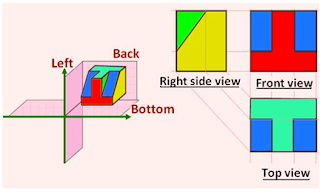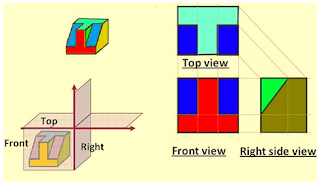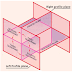## First Angle  and Third Angle Projection Methods | Engineering Drawing

To provide details of 2D drawing of any 3D object, two main types of projections are used and they are First Angle projection and Third Angle projection.
A collection of 2D drawings of any 3D object is represented with the help of orthographic projection. The orthographic projection consists of 6 views (Front, Back, Top, Bottom, Right, Left) called as principle views. Among these six orthographic views front view, right view and top view are the most commonly used to represent the orthographic projection of any object.

#### Method of Projection

Now, we know there are basically four quadrants. Hence to get the projections, we divide the object into four quadrants. The first quadrant represents the First Angle projection of the object while the third quadrant represents the Third Angle projection of the object. The principle projection planes and quadrants used to create 2D drawings can be seen in fig. 1.Fig. 1. Principle Projection Planes and Quadrants

Now, let us see the first angle and third angle projection in detail.

### First Angle Projection

In first angle projection, the object is placed in between the plane of projection and the observer as shown in fig.2.Fig.2. An object in between Plane of projection and observer

As explained above, in first angle projection, an object is placed in the first quadrant. The views are obtained by projecting the image of an object in the respective plane. Here you have to note down that the right-hand side view is projected on the plane placed at the left of the object. After projecting the images on the respective planes, the bottom plane and the left plane is unfolded onto the front view i.e. left plane is unfolded towards the left side to get Right Hand Side view on the left side of the front view. Similarly, the bottom plane is unfolded towards the bottom to obtain the Top view placed below the front view as shown in fig. 3.Fig.3. First Angle Method

### Third Angle Projection

In third angle projection, the plane of projection is placed in between the object and the observer as shown in fig.4.Fig.4. Plane of projection in between object and observer
In this type of projection, the object is placed in the third quadrant. The views are obtained by projecting the image on the respective plane as shown in fig.5.Fig.5. Third Angle Projection

## Symbols of First Angle Projection and Third Angle Projection

As per BIS standard, drawing symbol for first angle projection and third angle projection are shown in fig.6.Fig.6. Projection Symbol

## The difference between First Angle projection and Third Angle Projection

Due to an increase in the complication in drawings, second angle projection and fourth angle projection are not used. The difference between first angle projection and third angle projection is given below.

First Angle and Third Angle Projection Methods | Engineering DrawingReviewed by Unknown on 16:12 Rating: 5